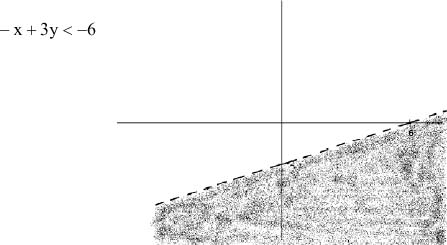Slope-Intercept Form

Slope-Intercept Form

When graphing a line, it is often helpful to write the equation in slope-intercept form, y = mx + b, where m is the slope of the line, and b is the y-intercept.

The slope of a line may also be determined when two points on the line are known.  The slope is calculated as the change in the y values over the change in the x values.

Linear inequalities may be graphed in the same way as lines.  Graph the equation as if it had an equal sign.  Use a dashed line for < or >. Use a solid line for < or >.  Test a point not on the line to check whether it is a solution of the inequality.  If the test point is a solution, shade its region. If the test point is not a solution, shade the other region.

Practice

1.    Find the x and y-intercepts, slope and graph of 6x + 5y = 30.

2.    Find the x and y-intercepts, slope and graph of x = 3.

3.    Find the x and y-intercepts, slope and graph of y = -4.

4.    Write in slope-intercept form the line that passes through the points (4, 6) and (-4, 2).

5.    Write in slope-intercept form the line perpendicular to the graph of 4x - y = -1 and containing the point (2, 3).

6.    Graph the solution set of x - y > 2.

7.    Graph the solution set of -x + 3y < - 6.

1.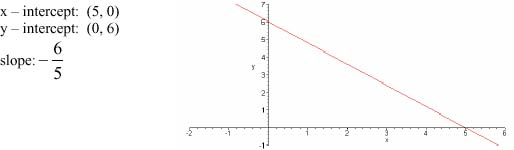2.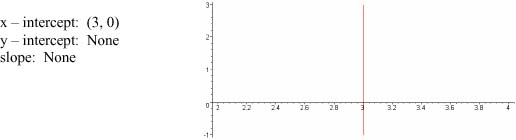3.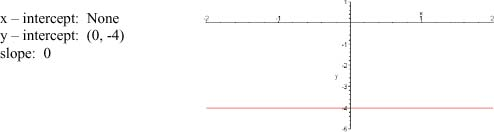4.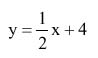5.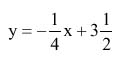6.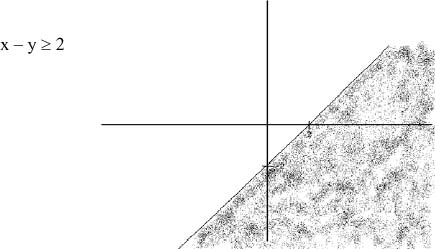7.